## RSA Encryption

A Public-Key Cryptography Algorithm which uses Prime Factorization as the Trapdoor Function. Define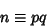(1)

for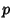and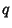Primes. Also define a private keyand a public keysuch that(2)(3)

whereis the Totient Function.

Let the message be converted to a number. The sender then makesandpublic and sends(4)

To decode, the receiver (who knows) computes(5)

since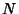is an Integer. In order to crack the code,must be found. But this requires factorization ofsince(6)

Bothandshould be picked so thatand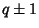are divisible by large Primes, since otherwise the Pollard p-1 Factorization Method or Williams p+1 Factorization Method potentially factoreasily. It is also desirable to havelarge and divisible by large Primes.

It is possible to break the cryptosystem by repeated encryption if a unit of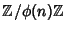has small Order (Simmons and Norris 1977, Meijer 1996), whereis the Ring of Integers between 0 andunder addition and multiplication (mod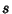). Meijer (1996) shows that almost'' every encryption exponentis safe from breaking using repeated encryption for factors of the form(7)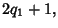(8)

where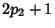(9)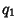(10)

and,,,,, and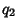are all Primes. In this case,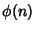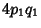(11)(12)

Meijer (1996) also suggests thatandshould be of order 1075.

Using the RSA system, the identity of the sender can be identified as genuine without revealing his private code.

References

Honsberger, R. Mathematical Gems III. Washington, DC: Math. Assoc. Amer., pp. 166-173, 1985.

Meijer, A. R. Groups, Factoring, and Cryptography.'' Math. Mag. 69, 103-109, 1996.

Rivest, R. L. Remarks on a Proposed Cryptanalytic Attack on the MIT Public-Key Cryptosystem.'' Cryptologia 2, 62-65, 1978.

Rivest, R.; Shamir, A.; and Adleman, L. A Method for Obtaining Digital Signatures and Public Key Cryptosystems.'' Comm. ACM 21, 120-126, 1978.

RSA Data Security.A Security Dynamics Company. http://www.rsa.com.

Simmons, G. J. and Norris, M. J. Preliminary Comments on the MIT Public-Key Cryptosystem.'' Cryptologia 1, 406-414, 1977.